Question

# A 249−mL benzene solution containing 2.35 g of an organic polymer has an osmotic pressure of...

A 249−mL benzene solution containing 2.35 g of an organic polymer has an osmotic pressure of 7.91 mm Hg at 19°C. Calculate the molar mass of the polymer.

We Have

Osmotic Pressure P = 7.91 mm Hg = 7.91/760 = 0.0104 atm

Temperature T = 190 C = 19 + 273 = 292 K

Volume = 249 mL = 0.249 L

mass of polymer = 2.35 g

R = Ideal Gas Constant = 0.082057 L atm mol-1 K-1

C = Molar Concentration = ?

So We know the formula

Osmotic Pressure = CRT

So

0.0104 atm = C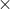0.082057 L atm mol-1 K-1292 K

C = 0.0104 atm/ 23.96 atm mol-1 = 4.3410-4 M

Now Lets Calculate the number of moles by given volume

Moles of Polymer = 4.3410-4 M0.249 L = 1.0810-4 mol

From this we can get Molar mass now

Molar mass of Polymer = mass of polymer / moles of Polymer = 2.35 g / 1.0810-4 mol

= 2.17104 g/mol

So Our Answer is = 2.17104 g/mol

#### Earn Coins

Coins can be redeemed for fabulous gifts.

Similar Homework Help Questions
• ### A 279−mL benzene solution containing 2.79 g of an organic polymer has an osmotic pressure of...

A 279−mL benzene solution containing 2.79 g of an organic polymer has an osmotic pressure of 8.19 mm Hg at 11°C. Calculate the molar mass of the polymer.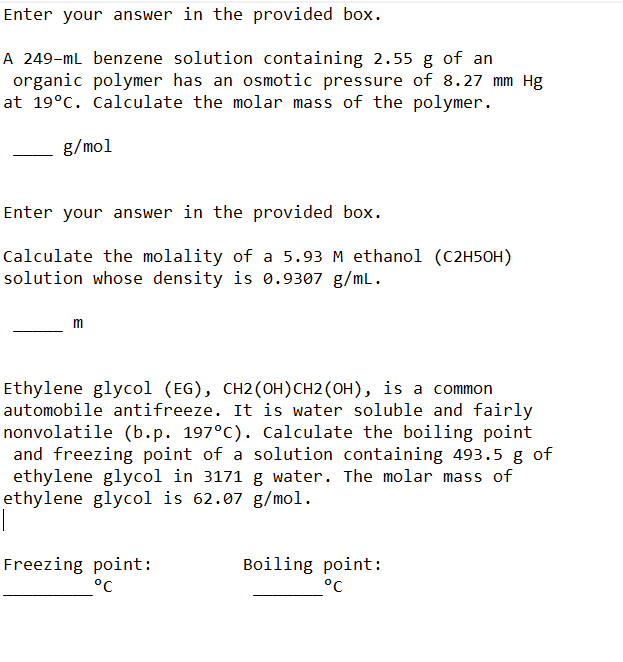Please help I will rate :) Enter your answer in the provided box. A 249-ml benzene solution containing 2.55 g of an organic polymer has an osmotic pressure of 8.27 mm Hg at 19°C. Calculate the molar mass of the polymer. - g/mol Enter your answer in the provided box. Calculate the molality of a 5.93 M ethanol (C2H5OH) solution whose density is 0.9307 g/mL. Ethylene glycol (EG), CH2(OH)CH2 (OH), is a common automobile antifreeze. It is water soluble and...

• ### The osmotic pressure of a solution containing 22.7 mg of an unknown protein in 50.0 mL...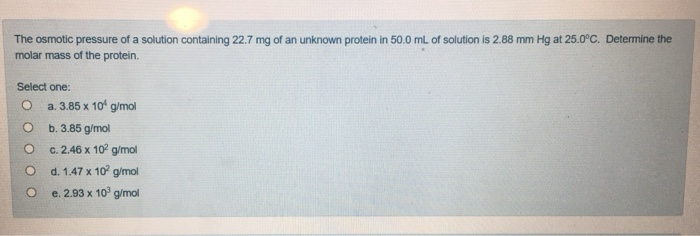The osmotic pressure of a solution containing 22.7 mg of an unknown protein in 50.0 mL of solution is 2.88 mm Hg at 25.0°C. Determine the molar mass of the protein. Select one: O a. 3.85 x 10 g/mol O b.3.85 g/mol O c. 2.46 x 10 g/mol O d. 1.47 x 10 g/mol e. 2.93 x 109 g/mol

• ### A) Calculate the osmotic pressure of a solution containing 16.75 mg of hemoglobin in 15.7 mL...

A) Calculate the osmotic pressure of a solution containing 16.75 mg of hemoglobin in 15.7 mL of solution at 22 ∘C . The molar mass of hemoglobin is 6.5×104 g/mol. B) A 2.400×10−2M solution of NaCl in water is at 20.0∘C. The sample was created by dissolving a sample of NaCl in water and then bringing the volume up to 1.000 L. It was determined that the volume of water needed to do this was 999.4 mL . The density...

• ### Calculate the osmotic pressure of a solution containing 19.50 mg of hemoglobin in 13.1 mL of...

Calculate the osmotic pressure of a solution containing 19.50 mg of hemoglobin in 13.1 mL of solution at 32 ∘C . The molar mass of hemoglobin is 6.5×104 g/mol. Express your answer in atmospheres.

• ### In a laboratory experiment, a student found that a 158-mL aqueous solution containing 2.059 g of...

In a laboratory experiment, a student found that a 158-mL aqueous solution containing 2.059 g of a compound had an osmotic pressure of 16.9 mm Hg at 298 K. The compound was also found to be nonvolatile and a nonelectrolyte. What is the molar mass of this compound?

• ### Calculate the osmotic pressure in torr of a solution containing 18.75 mg of hemoglobin in 15.0...

Calculate the osmotic pressure in torr of a solution containing 18.75 mg of hemoglobin in 15.0 mL of solution at 25.0oC. The molar mass of hemoglobin is 6.5 x 104g/mol.

• ### Polyethylene is a synthetic polymer or plastic with many uses. 1.44 g of a polyethylene sample...

Polyethylene is a synthetic polymer or plastic with many uses. 1.44 g of a polyethylene sample was dissolved in enough benzene to make 100. mL of solution, and the osmotic pressure was found to be 1.86 torr at 25oC. What is the approximate molar mass of the polyethylene?

• ### Practice Exercise 12.25 g of the protein (molar mass = 235,000 g mol What is the...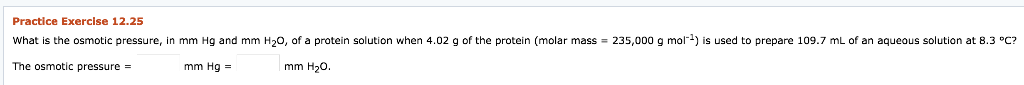Practice Exercise 12.25 g of the protein (molar mass = 235,000 g mol What is the osmotic pressure, mm Hq and mm H20, of a protein solution when 4.02 is used to prepare 109.7 mL of an aqueous solution at 8.3 °C? mm H20 The osmotic pressure = mm Hg = Practice Exercise 12.25 g of the protein (molar mass = 235,000 g mol What is the osmotic pressure, mm Hq and mm H20, of a protein solution when 4.02...

• ### What is the vapor pressure for an aqueous sucrose (C12H22011) solution containing 34.2 g of sucrose...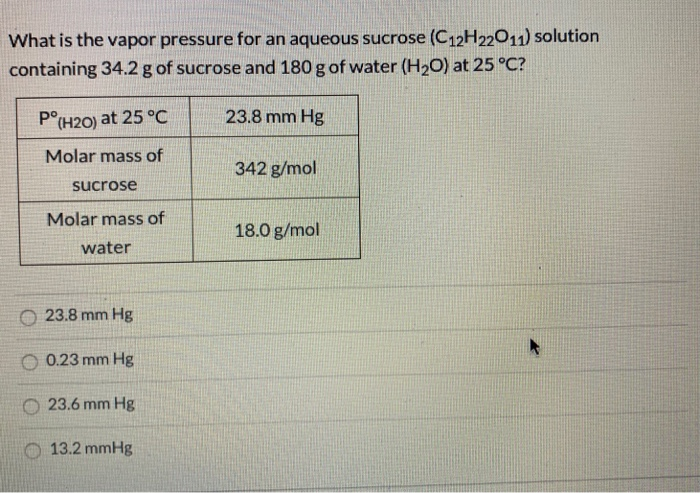What is the vapor pressure for an aqueous sucrose (C12H22011) solution containing 34.2 g of sucrose and 180 g of water (H2O) at 25°C? Pº(H20) at 25°C 23.8 mm Hg Molar mass of 342 g/mol sucrose Molar mass of 18.0 g/mol water 23.8 mm Hg O 0.23 mm Hg 23.6 mm Hg 13.2 mmHg